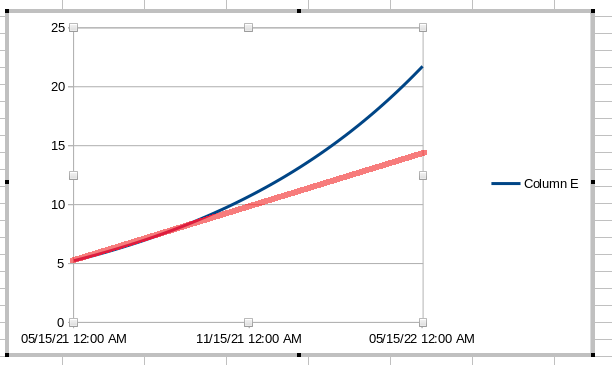Comment on page

# The Jar APY - The Math Behind Compounding

• Nerd Math Below
Pickle Finance lists APY statistics for all of their jars. These calculations are always based on the current advertised rates of the underlying liquidity pools, lending platforms, or other protocols, (also known as the Base Rate), as well as the expected reward token emissions and their current prices as determined by a price oracle like CoinGecko.
Since Pickle Finance is a compounding service, and because Pickle Finance only compounds reward tokens, Pickle Finance needs to mathematically calculate the advertised reward token rate after compounding it. This calculation includes an assumption: Pickle Finance will compound the pool daily. The calculation also reflects that Pickle Finance will be collecting 20% of the harvested rewards.
To convert an APR to an APY, Pickle Finance will perform the following calculation: APY = (baseAPR + (((1+(0.8*rewardAPR/365))^365)-1)) For example, a strategy with a 10% base yield, and a 100% reward token yield, should yield the following calculation. Note that we use 0.1 in place of 10% and 1.00 in place of 100%. APY = (0.1 + (((1+(0.8*1.00/365))^365)-1)) = 1.3236 To convert this to a percentage, multiply by 100. This strategy should return 132.36%. Using Pickle has increased your APR from 110% to 132% after fees. The above calculation assumes daily compounding of your strategy. Some strategies do not compound daily, despite our best efforts. Either the pools do not have enough money in them, or the strategy does not return enough rewards relative to gas costs to justify daily compounding. This is not a bad thing. For lower and medium yielding strategies the difference between daily or weekly compounding is minimal. To run the calculation again, but with a different frequency for compounding, you should adjust the chosen formula to the following: APY = (baseAPR + (((1+(0.8*rewardAPR/compoundsPerYear))^compoundsPerYear)-1))
If we assume a strategy is only compounded once a month, we can run the calculation as follows: APY = (0.1 + (((1+(0.8*1.00/12))^12)-1)) = 1.269 Using the same example as above (a 10% base rate with a 100% rewards rate), we come to the answer of 126.9%.

### Compounding Takes Time To Show Significant Results

• Compounding is slow
• Compounding looks linear, until it doesn’t.
One user complained that the growth of his pool appeared linear and not exponential. It is important to recognize that compound growth does not take place overnight, but rather is the result of a slow process that, like gravity, doesn’t stop and gets more powerful the longer you are affected by it. The chart below of a jar expecting 270+% growth over a year demonstrates that process:For the first five or six months, an exponential compounding (blue) will be difficult to distinguish from linear growth (red). However, once the two diverge, the compounding process speeds up at an increasing rate. Exponential growth requires patience to see results, even for very high APY jars.